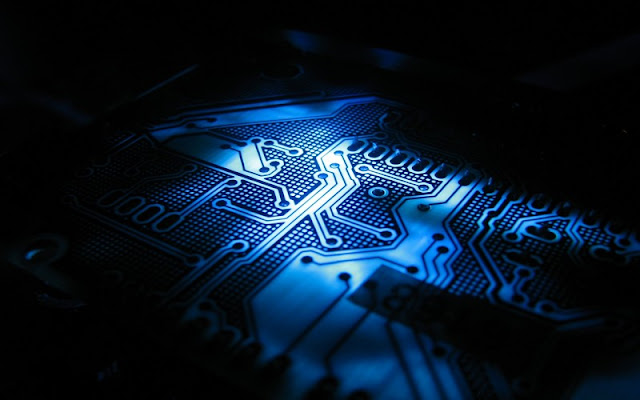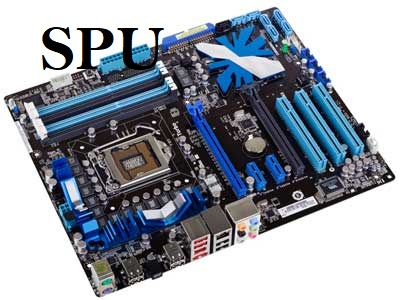# Different types of bit computer systems

Types of computer systems: in this post, we are going to learn about the different types of computer systems. we are gone to look at the bit systems and see how our computer becomes more powerful by time by time.

So, I tell you one thing that is in our computer system real magic comes with our processing units.

we already learned about the ones and notes which are the language of our computer system. Our processing units become more powerful and evaluate because they composed more once and not at a single time and giving us more range.So let's understand how our computer becomes more powerful day by day. and to understand this we need to first look at the different types of bit computer systems.

## we have Four types of computer systems

• 8 Bit computer system
• 16 Bit computer system
• 32 Bit computer system
• 64 Bit computer system

### 8 Bit System

What we want to do let's find out possible combinations number of 1 and 0 there is.

So we work with the 8-bit system and the range of the 8-bit system is 256(including zero) possibilities.
0 to 255### 16 Bit System

the range of the 16-bit system is 0 to 65,535. and we have 65, 536 combinations of zeros and ones.
0 to 65,535

### 32 Bit System

in the 32 Bit computer system we have 0 to 4,294,967,295 combinations of zeros and ones. we have a range of 32 Bit computer systems is 4,294,967,296.
0 to 4,294,967,296

### 64 Bit computer system

in 64 Bit computer system we have 0  to 18,446,744,073,709,551,615 combinations of zeros and ones. we have a range of 64 Bit computer systems is 18,446,744,073,709,551,616.
0 to 18,446,744,073,709,551,616
In every computer system, we have GPU, SPU, and CPU. if you don't know what is GPU, SPU and CPU then read my previous post. you will get the link at the end of the post.

#### Graphical processing unit

When we sand 256 possible binary numbers to the GPU with
0  = Black color             255 = White color
and any possible number between two points produced a color like green, red.

So, we have 256 possible colors. and in other bit systems like 16, 32 and 64 bit systems we have more combinations of colors. the more combinations of colors we have the more powerful our computer becomes.#### Sound processing unit

In the 8-bit system, we have 256 possible binary combinations and 256 possible sound waves. but the 256 sound waves is not sufficient to hear. So our sounds get a little bit tinny. but in 16, 32 and 64-bit computer systems we have more combinations of sound waves. so we have the pure quality of sound.How the SPU works?

#### Core processing unit

In the 8-bit system. we have only 255 maximum combinations to produce output and a maximum 255 number we get. so we have a maximum of 255 pixels to display. but in 16, 32 and 64-bit computer systems we have more combinations of zeros and once. so we have more pixels to work on. and the more pixels we have the more our CPU becomes powerful.So as well as in 16, 32, and 64 bit systems. the output depends on an only range of bit system.

Example
As an example, the range of the 16-bit system is 0 to 65,535. so we get 65,536 possible bases two numbers.
So, in GPU we get 65,535 colors, sound waves and mathematical levels in the 16-bit system.